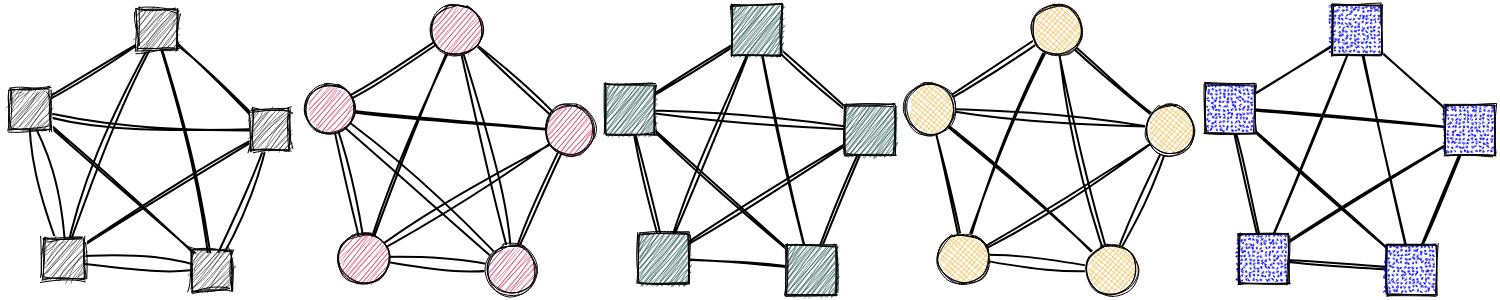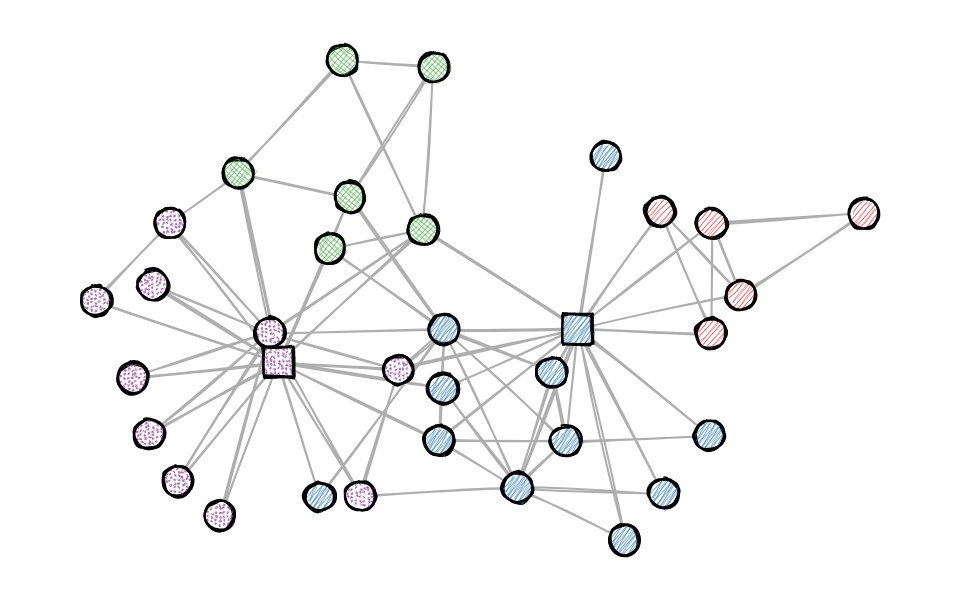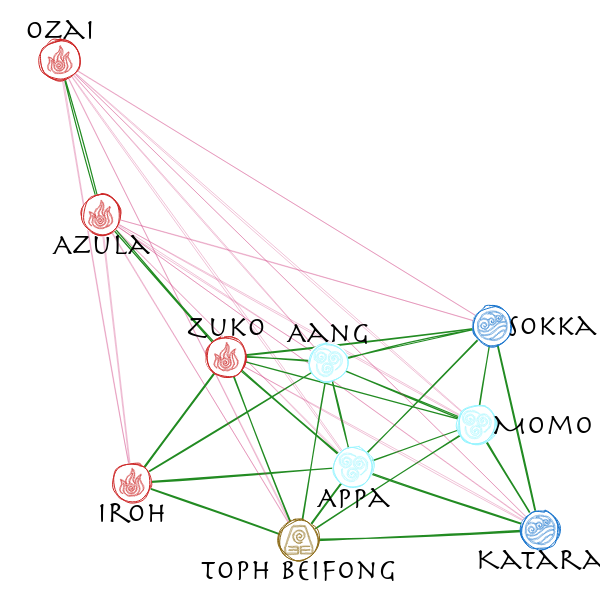# roughnet

An R package to draw sketchy networks

2020-06-25

Using the java script library rough.js to draw sketchy, hand-drawn-like networks.
(Checkout ggrough for turning general ggplot objects into sketchy drawings)## Installation

You can install the developers version of roughnet with:

``````# install.packages("remotes")
remotes::install_github("schochastics/roughnet")``````

## Example

The package currently only works with {{igraph}} objects by setting styling elements as vertex/edge attributes.

``````library(roughnet)
library(igraph)

g <- make_graph("Zachary")
V(g)\$shape <- "circle"
V(g)\$shape[c(1,34)] <- "rectangle"
V(g)\$fill <- c("#E41A1C", "#377EB8", "#4DAF4A", "#984EA3")[membership(cluster_louvain(g))]
V(g)\$fillstyle <- c("hachure", "zigzag", "cross-hatch", "dots")[membership(cluster_louvain(g))]
V(g)\$color <- "black"
V(g)\$size <- 30
V(g)\$stroke <- 2
E(g)\$color <- "#AEAEAE"
roughnet(g,width = 960,height = 600)``````You can save the plot with the function `save_roughnet()` (needs {{pagedown}} to be installed.)

``````# install.packages("pagedown")
p <- roughnet(g,width = 960,height = 600)
save_roughnet(p,"karate.png")``````

## Extended Example

The package also allows to place labels in or around vertices and implements some custom vertex shapes.

``````# install.packages(c("signnet","graphlayouts"))
library(signnet)
library(graphlayouts)
library(dplyr)

data("avatar") # Allies/Enemies relations in Avatar: The Last Airbender

main <- induced_subgraph(avatar,which(V(avatar)\$main)) #only use the main characters

#calculate layout
w <- ifelse(E(main)\$sign==1,3,1)
xy <- layout_with_stress(main,weights = 1/w)

V(main)\$x <- xy[,1]
V(main)\$y <- xy[,2]

V(main)\$fill <- case_when(V(main)\$affiliation=="earth kingdom"~"#8B6914",
V(main)\$affiliation=="fire nation"~"#CD2626",
V(main)\$affiliation=="water tribe"~"white",
TRUE~"grey"
)

V(main)\$color <- case_when(V(main)\$affiliation=="earth kingdom"~"#8B6914",
V(main)\$affiliation=="fire nation"~"#CD2626",
V(main)\$affiliation=="water tribe"~"#1874CD",
TRUE~"grey"
)

V(main)\$shape <- case_when(V(main)\$affiliation=="earth kingdom"~"earth",
V(main)\$affiliation=="fire nation"~"fire",
V(main)\$affiliation=="water tribe"~"water",